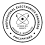### ECE REVIEWER MATHEMATICS

SYLLABI FOR THE ELECTRONICS ENGINEER (ECE) LICENSURE EXAMINATION

I. MATHEMATICS (20%)

1. Algebra & General Mathematics
_ Algebraic functions
_ Theory of equations
_ Factorization and algebraic functions
_ Ratio, proportion and variation
_ Matrix theory
_ Arithmetic and geometric progressions
_ Equations and inequalities
_ Complex number system
_ Polynomials
_ Mathematical induction
_ Logic and probability
_ Statistics

2. Geometry
_ Lines and planes
_ Plane figures
_ Application of Cavalier’s, Pappus and Prismodial Theorems
_ Coordinates in space
_ Mensuration
_ Plane geometry
_ Solid geometry
_ Spherical geometry
_ Analytical geometry

3. Trigonometry
_ Logarithmic principles
_ Trigonometric functions
_ Fundamental trigonometric identities
_ Solutions of right and oblique triangles
_ Applications of terrestrial mensuration
_ Area, perimeter and centroid of plane figures
_ Polar coordinates
_ Spherical trigonometry

4. Calculus
_ Complex variables
_ Derivatives and applications
_ Integration and applications
_ Transcendental functions
_ Partial derivatives
_ Higher derivatives
_ Indeterminate forms
_ Multiple integrals
_ Differential equations

5. Mathematics Laws, Terms and Theories
_ Laws, theories and other rules relative to the fields of mathematics

ECE REVIEWER

1.Hi Sir, I am a graduate of BS ECE 2005. Balak ko po sana mag take ng exam ulit kaso parang ang dame na po pagbabago. Ano na po scope ng exam ngayon para sa BS ECE??

1.http://www.electronicspectrum.com/2012/03/ece-licensure-exam-syllabi.html

2.sir my solution ba kau nung mga pronlem solving poh salamat

1.i think iilan sa mga questions dito sa Math ay may solution. Kung wala man, esearch mo lang sa internet ang mga formulas. Maganda yon nag sesearch at nagcocompute ng sarili para mapractice.

3.sir jandee pede ba magpatutor sa inyo balak ko po xe magtake sa april 2018 nhrapan na po xe me sa computation sa math and geas salamat

1.mahirap ang magtutor thru online lalo na pag computation. I suggest na mag enroll ka sa review center.

4.Hi po. Condi po ako sa Math nung April 2015, pwede ko pa po ba yun itake sa april? I forgot kung ilang years yung validity nun e. Thank you :)

1.yes, pwede.

The removal examination is a one-time opportunity only, but may be taken by the candidate at any time.

Kaya marami ka time na paghandaan ang MATH. Kung feel mo di pa enough ang pag-aaral by April, take it on the next exam schedule.

Good Luck!